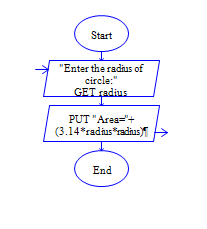# C Program to find area of Circle (Use Constant)

Get radius of circle and find area of circle.

Sample Input 1:

4

Sample Output 1:

50.24

#### Flow Chart DesignStrongly recommended to Solve it on your own, Don't directly go to the solution given below.

#include<stdio.h> int main() { //write your code here }

#### Program or Solution

``` #include<stdio.h> int main() { const float pi=3.14; int radius; float area; printf("Enter Radius:"); scanf("%d",&radius); area=pi * (radius * radius); printf("Area of Circle: %.2f",area); return 0; } ```

#### Program Explanation

Get radius of a circle (using scanf statement)